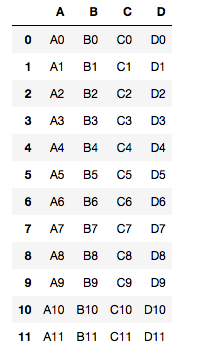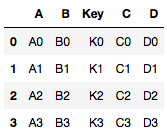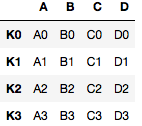# Python | Merge, Join and Concatenate DataFrames using Panda

A dataframe is a two-dimensional data structure having multiple rows and columns. In a dataframe, the data is aligned in the form of rows and columns only. A dataframe can perform arithmetic as well as conditional operations. It has mutable size.

Below is the implementation using Numpy and Pandas.

Modules needed:

```import numpy as np
import pandas as pd
```

Code #1 : DataFrames Concatenation
`concat()` function does all of the heavy lifting of performing concatenation operations along an axis while performing optional set logic (union or intersection) of the indexes (if any) on the other axes.

 `# Python program to concatenate ` `# dataframes using Panda ` ` `  `# Creating first dataframe ` `df1 ``=` `pd.DataFrame({``'A'``: [``'A0'``, ``'A1'``, ``'A2'``, ``'A3'``], ` `                    ``'B'``: [``'B0'``, ``'B1'``, ``'B2'``, ``'B3'``], ` `                    ``'C'``: [``'C0'``, ``'C1'``, ``'C2'``, ``'C3'``], ` `                    ``'D'``: [``'D0'``, ``'D1'``, ``'D2'``, ``'D3'``]}, ` `                    ``index ``=` `[``0``, ``1``, ``2``, ``3``]) ` ` `  `# Creating second dataframe ` `df2 ``=` `pd.DataFrame({``'A'``: [``'A4'``, ``'A5'``, ``'A6'``, ``'A7'``], ` `                    ``'B'``: [``'B4'``, ``'B5'``, ``'B6'``, ``'B7'``], ` `                    ``'C'``: [``'C4'``, ``'C5'``, ``'C6'``, ``'C7'``], ` `                    ``'D'``: [``'D4'``, ``'D5'``, ``'D6'``, ``'D7'``]}, ` `                    ``index ``=` `[``4``, ``5``, ``6``, ``7``]) ` ` `  `# Creating third dataframe ` `df3 ``=` `pd.DataFrame({``'A'``: [``'A8'``, ``'A9'``, ``'A10'``, ``'A11'``], ` `                    ``'B'``: [``'B8'``, ``'B9'``, ``'B10'``, ``'B11'``], ` `                    ``'C'``: [``'C8'``, ``'C9'``, ``'C10'``, ``'C11'``], ` `                    ``'D'``: [``'D8'``, ``'D9'``, ``'D10'``, ``'D11'``]}, ` `                    ``index ``=` `[``8``, ``9``, ``10``, ``11``]) ` ` `  `# Concatenating the dataframes ` `pd.concat([df1, df2, df3]) `

Output:Code #2 : DataFrames Merge
Pandas provides a single function, merge(), as the entry point for all standard database join operations between DataFrame objects.

 `# Python program to merge ` `# dataframes using Panda ` ` `  `# Dataframe created ` `left ``=` `pd.DataFrame({``'Key'``: [``'K0'``, ``'K1'``, ``'K2'``, ``'K3'``], ` `                    ``'A'``: [``'A0'``, ``'A1'``, ``'A2'``, ``'A3'``], ` `                    ``'B'``: [``'B0'``, ``'B1'``, ``'B2'``, ``'B3'``]}) ` ` `  `right ``=` `pd.DataFrame({``'Key'``: [``'K0'``, ``'K1'``, ``'K2'``, ``'K3'``], ` `                      ``'C'``: [``'C0'``, ``'C1'``, ``'C2'``, ``'C3'``], ` `                      ``'D'``: [``'D0'``, ``'D1'``, ``'D2'``, ``'D3'``]}) ` `                       `  `# Merging the dataframes                       ` `pd.merge(left, right, how ``=``'inner'``, on ``=``'Key'``) `

Output:Code #3 : DataFrames Join

 `# Python program to join ` `# dataframes using Panda ` ` `  `left ``=` `pd.DataFrame({``'A'``: [``'A0'``, ``'A1'``, ``'A2'``, ``'A3'``], ` `                    ``'B'``: [``'B0'``, ``'B1'``, ``'B2'``, ``'B3'``]}, ` `                    ``index ``=` `[``'K0'``, ``'K1'``, ``'K2'``, ``'K3'``]) ` ` `  `right ``=` `pd.DataFrame({``'C'``: [``'C0'``, ``'C1'``, ``'C2'``, ``'C3'``], ` `                      ``'D'``: [``'D0'``, ``'D1'``, ``'D2'``, ``'D3'``]}, ` `                      ``index ``=` `[``'K0'``, ``'K1'``, ``'K2'``, ``'K3'``]) ` `                       `  `# Joining the dataframes                       ` `left.join(right) `

Output:My Personal Notes arrow_drop_upCheck out this Author's contributed articles.

If you like GeeksforGeeks and would like to contribute, you can also write an article using contribute.geeksforgeeks.org or mail your article to contribute@geeksforgeeks.org. See your article appearing on the GeeksforGeeks main page and help other Geeks.

Please Improve this article if you find anything incorrect by clicking on the "Improve Article" button below.

Article Tags :

1

Please write to us at contribute@geeksforgeeks.org to report any issue with the above content.